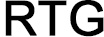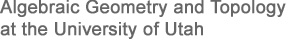# Faculty

## Direction

Ken Bromberg (PI, Director)
Tommaso de Fernex (Co-PI, Co-Director)
Aaron Bertram (Co-PI, In-House Consultant)

## RTG Group

Aaron Bertram - Works in algebraic geometry, specifically on questions about moduli spaces related to mirror symmetry. Current interests include stable maps and Gromov-Witten theory, derived categories and Bridgeland's stability conditions and the relationship between classical Riemann surface theory and tropical curves (i.e., metric graphs).

Mladen Bestvina - Works in geometric group theory. Of particular interest is the geometry of Outer Space (the space associated to Out(Fn)). Other interests include: mapping class groups and Teichmuller space, asymptotic dimension, bounded cohomology, limit groups.

Ken Bromberg - Works on hyperbolic geometry and deformation spaces of Kleinian groups. Particularly interested in the "bumping" phenomena leading to non-local connectivity of deformation spaces. Other interests: geometric group theory, actions on quasi-trees, asymptotic dimension.

Jon Chaika - Works on ergodic theory, interval exchange transformations and flows on flat surfaces. Particularly interested in diophantine approximation and also non-unique ergodicity. Other interests include properties of and actions on the parametrizing space of interval exchanges and flat surfaces, diophantine approximation in abstract settings and isomorphism problems.

Tommaso de Fernex - Works in algebraic geometry, with focus on problems in birational geometry of higher dimensional varieties and the minimal model program. Of particular interest are topics in singularity theory such as multiplier ideals, spaces of arcs, and valuation theory.

Christopher Hacon - Works in algebraic geometry. He is especially interested in higher dimensional birational geometry and questions related to the minimal model program (in zero and positive characteristics). In particular he studies global properties such as the behavior of pluricanonical maps and local questions concerning invariants of singularities (log canonical thresholds and minimal log discrepancies).

Srikanth Iyengar - Main research interest is commutative ring theory; more precisely, in its homological aspects like free resolutions (finite and infinite), structure of morphisms, Hochschild and Andre-Quillen (co)homology, and in various triangulated categories linked to commutative rings. He often borrows techniques from algebraic topology and representation theory and has sometimes managed to return the favor.

Yuan-Pin Lee - Works on algebraic geometry and mathematical physics, with emphasis on Gromov-Witten theory and its relations with and applications to algebraic cobordism, birational geometry, K-theory, integrable systems, mirror symmetry, moduli of curves and variation of Hodge structures.

Stefan Patrikis - Works in number theory, arithmetic geometry, and representation theory, with an emphasis on questions arising out of the Langlands program. Current work focuses on Galois representations: deformation theory, monodromy groups and independence-of-l questions, and their motivic origin (the Fontaine-Mazur conjecture).

Karl Schwede - Works in algebraic geometry and commutative algebra. He is especially interested in singularities, their relation to the minimal model program and characteristic p methods. Some particular topics of work include Frobenius splittings, multiplier ideals, Du Bois singularities and tight closure theory.

Anurag Singh - Works in commutative algebra, specifically on questions related to local cohomology theory, tight closure theory, and the local homological conjectures. Current projects include the study of local cohomology in mixed characteristic (i.e., in the arithmetic situation), using combinations of prime characteristic methods and differential operators in characteristic zero.

Domingo Toledo - Interested in topological and geometric properties of moduli spaces that appear in algebraic geometry. Another interest: existence of non-arithmetic lattices in complex hyperbolic spaces.

Kevin Wortman - Works in geometric group theory, particularly lattices in locally compact groups. Of particular interest are finiteness properties, isoperimetric functions, and quasi-isometric rigidity.